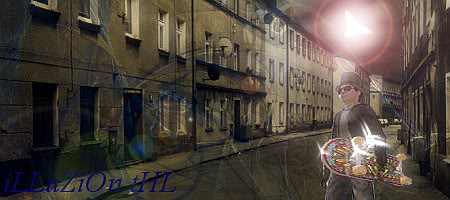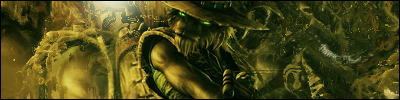tHL Forum-Site Currently Under ConstructiontHL Forum- :: Introduction Share

:)))))))))))))AuthorMessage
Eraser

Posts : 49
Join date : 2009-05-27Subject: :)))))))))))))Wed May 27, 2009 12:02 pm Hi I'm Eraser maybe you know me maybe you don't but hello lol.@@@@@@@@@@brb checking some site sectionsiLLuZiOn tHLPosts : 214
Join date : 2009-05-01Subject: Re: :)))))))))))))Thu May 28, 2009 6:13 pm Welcome to the site eraser_________________N1ck tHLPosts : 285
Join date : 2009-05-01Subject: Re: :)))))))))))))Thu May 28, 2009 7:39 pm Hey. Good to have you._________________Subject: Re: :))))))))))))):)))))))))))))